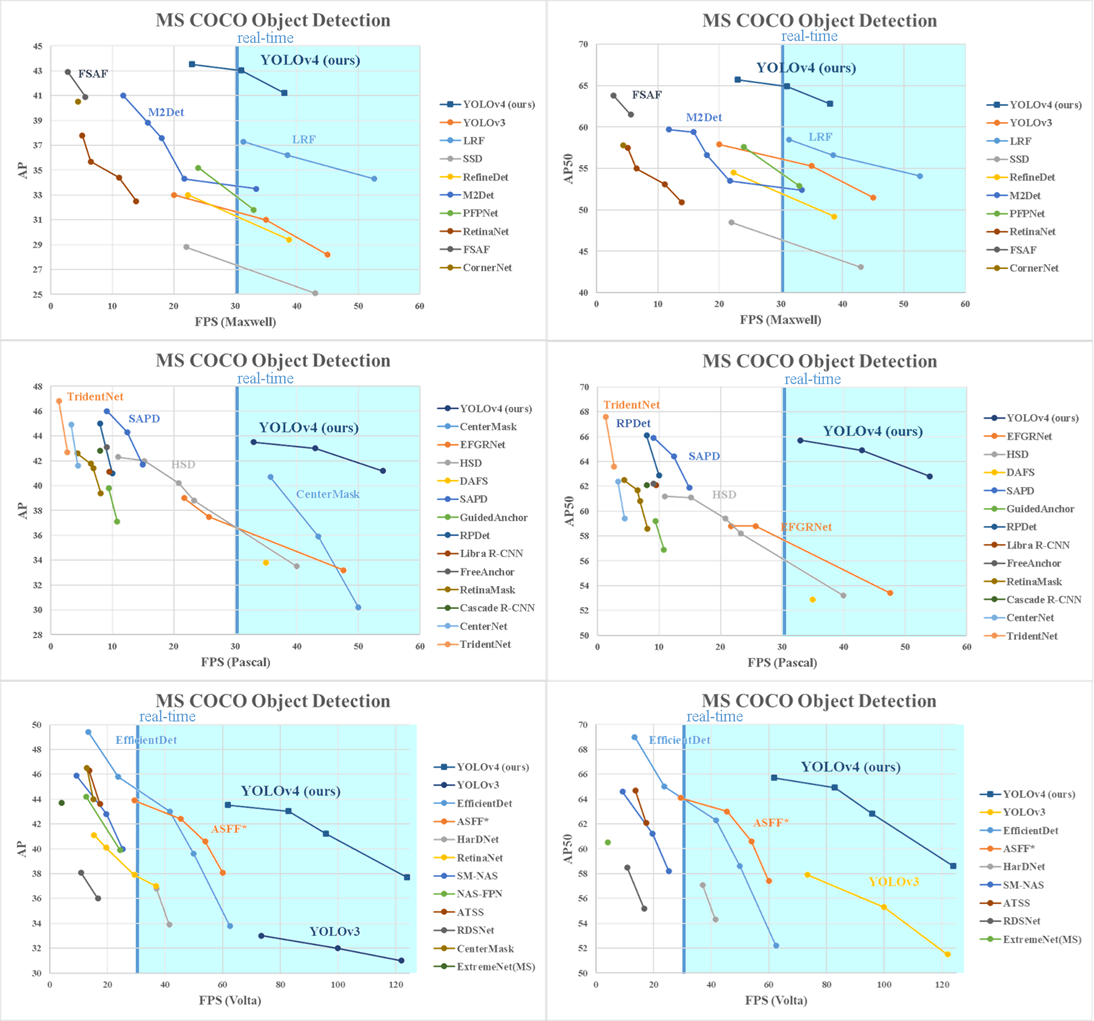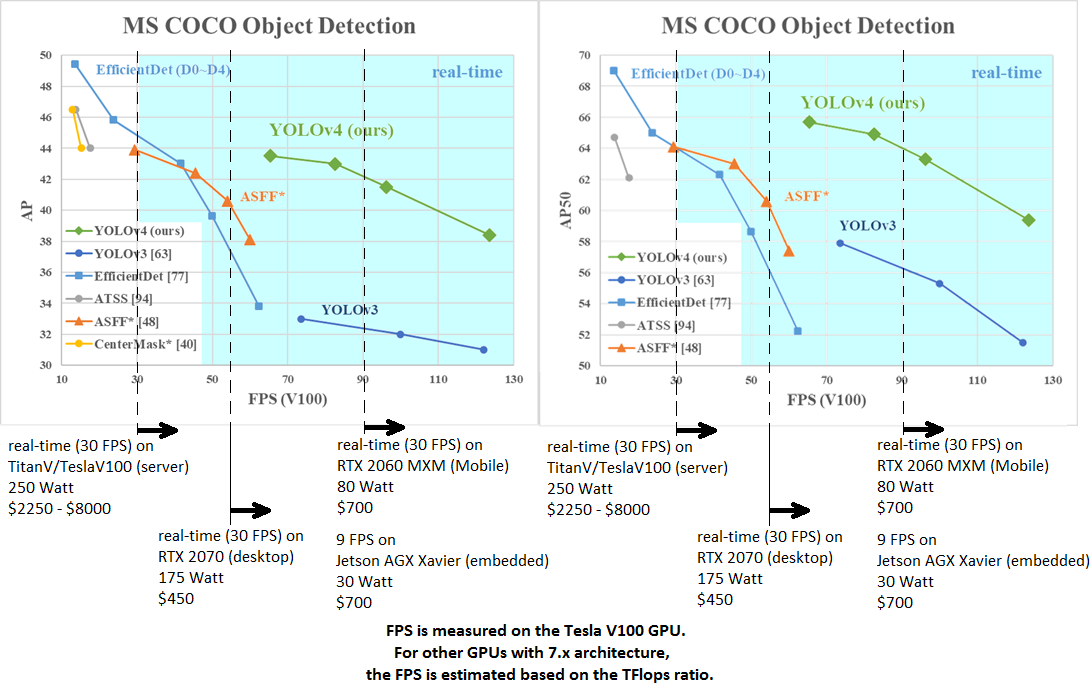# Feature-request: State-of-art Yolo v4 Detector

Feature-request: State-of-art Yolo v4 Detector

Many other features from Darknet were added previously.
But should be added at least new two features:

1. Mish-activation:
```float softplus(float x, float threshold = 20) {
if (x > threshold) return x;                // too large
else if (x < -threshold) return expf(x);    // too small
return logf(expf(x) + 1);
}

float mish_activation(float input) {
const float MISH_THRESHOLD = 20;
output = input * tanh( softplus(input, MISH_THRESHOLD) );
return output;
}```

1. Eliminate grid sensitivity – by using `scale_x_y=` parameter from `[yolo]` layer in cfg-file (by default use `scale_x_y=1.0` if the value is not set in cfg file)
we should use:
```const float x_tmp = logistic_activate(srcData[box_index + 0]) * scale_x_y - (scale_x_y - 1) / 2;
const float y_tmp = logistic_activate(srcData[box_index + 1]) * scale_x_y - (scale_x_y - 1) / 2;
dstData[box_index + 0] = (x + x_tmp)) / cols;
dstData[box_index + 1] = (y + y_tmp)) / rows;```

instead of

Lines 305 to 306
in
51a42c0

 dstData[box_index + 0] = (x + logistic_activate(srcData[box_index + 0])) / cols; dstData[box_index + 1] = (y + logistic_activate(srcData[box_index + 1])) / rows;

Comparison of detectors on Microsoft COCO dataset and Tesla V100 GPU:Full dicussion on github.com

## 1 possible answer(s) on “Feature-request: State-of-art Yolo v4 Detector”

1. Hi! PR #17185 with Yolo v4 support has been merged to 3.4 branch